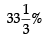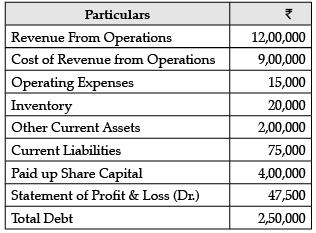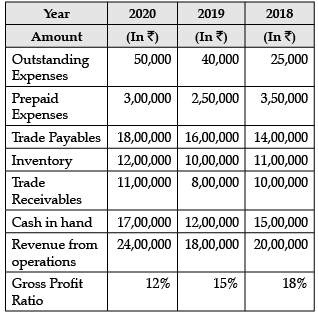Test: Accounting Ratios- Case Based Type Questions

# Test: Accounting Ratios- Case Based Type Questions

Test Description

## 12 Questions MCQ Test Accountancy Class 12 | Test: Accounting Ratios- Case Based Type Questions

Test: Accounting Ratios- Case Based Type Questions for Commerce 2023 is part of Accountancy Class 12 preparation. The Test: Accounting Ratios- Case Based Type Questions questions and answers have been prepared according to the Commerce exam syllabus.The Test: Accounting Ratios- Case Based Type Questions MCQs are made for Commerce 2023 Exam. Find important definitions, questions, notes, meanings, examples, exercises, MCQs and online tests for Test: Accounting Ratios- Case Based Type Questions below.
Solutions of Test: Accounting Ratios- Case Based Type Questions questions in English are available as part of our Accountancy Class 12 for Commerce & Test: Accounting Ratios- Case Based Type Questions solutions in Hindi for Accountancy Class 12 course. Download more important topics, notes, lectures and mock test series for Commerce Exam by signing up for free. Attempt Test: Accounting Ratios- Case Based Type Questions | 12 questions in 24 minutes | Mock test for Commerce preparation | Free important questions MCQ to study Accountancy Class 12 for Commerce Exam | Download free PDF with solutions
 1 Crore+ students have signed up on EduRev. Have you?
Test: Accounting Ratios- Case Based Type Questions - Question 1

### Read the following hypothetical extract of ABC Ltd. and answer the questions that follow: The following information are given:Trade Receivables Turnover Ratio 4 timesCurrent Liabilities ₹ 5,000Average Debtors ₹ 1,80,000Working Capital Turnover Ratio 8 timesCash Revenue from Operations 25% of Revenue from OperationsGross Profit RatioWhat will be the value of current assets?

Detailed Solution for Test: Accounting Ratios- Case Based Type Questions - Question 1 Working Capital = Current Assets – Current Liabilities

Current Assets = Working Capital + Current Liabilities

= ₹1,20,000 + ₹5,000

= ₹1,25,000

Test: Accounting Ratios- Case Based Type Questions - Question 2

### Read the following hypothetical extract of ABC Ltd. and answer the questions that follow: The following information are given:Trade Receivables Turnover Ratio 4 timesCurrent Liabilities ₹ 5,000Average Debtors ₹ 1,80,000Working Capital Turnover Ratio 8 timesCash Revenue from Operations 25% of Revenue from OperationsGross Profit RatioWhat is the revenue from operations?

Detailed Solution for Test: Accounting Ratios- Case Based Type Questions - Question 2 Trade Receivables Turnover ratio

= Credit Revenue from Operations / Average Trade Receivables

4 = Credit Revenue from Operations / 1,80,000

Credit Revenue from Operations = ₹1,80,000 × 4 = ₹ 7,20,000

Credit Revenue from Operations = 75% of Revenue from Operations

₹7,20,000 = 75% of Revenue from Operations Revenue from Operations = ₹9,60,000

Test: Accounting Ratios- Case Based Type Questions - Question 3

### Read the following hypothetical extract of ABC Ltd. and answer the questions that follow: The following information are given:Trade Receivables Turnover Ratio 4 timesCurrent Liabilities ₹ 5,000Average Debtors ₹ 1,80,000Working Capital Turnover Ratio 8 timesCash Revenue from Operations 25% of Revenue from OperationsGross Profit RatioWhat is the working Capital?

Detailed Solution for Test: Accounting Ratios- Case Based Type Questions - Question 3 Working Capital Turnover Ratio

= Revenue from Operations / Working Capital

8 = 9,60,000 /Working Capital

Working Capital = 9,60,000 / 8 = ₹ 1,20,000

Test: Accounting Ratios- Case Based Type Questions - Question 4

Read the following hypothetical extract of ABC Ltd. and answer the questions that follow: The following information are given:

Trade Receivables Turnover Ratio 4 times

Current Liabilities ₹ 5,000

Average Debtors ₹ 1,80,000

Working Capital Turnover Ratio 8 times

Cash Revenue from Operations 25% of Revenue from Operations

Gross Profit RatioWhat is the Gross Profit?

Detailed Solution for Test: Accounting Ratios- Case Based Type Questions - Question 4 Gross Profit = Gross Profit Ratio × Revenue from Operations

=of ₹9,60,000 = ₹3,20,000

Test: Accounting Ratios- Case Based Type Questions - Question 5

Consider the following data and answer the questions that follow:What is the working capital turnover ratio?

Detailed Solution for Test: Accounting Ratios- Case Based Type Questions - Question 5 Working Capital Turnover Ratio = Revenue from Operations / Working Capital

= ₹12,00,000 / ₹1,45,000 = 8.28 times

Test: Accounting Ratios- Case Based Type Questions - Question 6

Consider the following data and answer the questions that follow:What is the Operating ratio?

Detailed Solution for Test: Accounting Ratios- Case Based Type Questions - Question 6 Operating Ratio Cost of Revenue from Operations + Operating Expenses / Revenue from Operations x 100

= ₹9,00,000+ ₹15,000 / ₹12,00,000 x 100

= 76.25%

Test: Accounting Ratios- Case Based Type Questions - Question 7

Consider the following data and answer the questions that follow:What is the Debt to Equity Ratio?

Detailed Solution for Test: Accounting Ratios- Case Based Type Questions - Question 7

Debt to equity Ratio = Total Debt / Shareholders' Fund

= ₹2,50,000 / ₹4,00,000 = 0.63:1

Test: Accounting Ratios- Case Based Type Questions - Question 8

Consider the following data and answer the questions that follow:What is the quick ratio?

Detailed Solution for Test: Accounting Ratios- Case Based Type Questions - Question 8 Quick Ratio = Liquid Assets / Current Liabilities

= ₹2,00,000 / ₹ 75,000 = 2.67 : 1

Test: Accounting Ratios- Case Based Type Questions - Question 9Cost of Revenue from Operations for the year 2020 would be ______________.

Detailed Solution for Test: Accounting Ratios- Case Based Type Questions - Question 9 Cost of Revenue from Operations for the year 2020 would be ₹21,12,000.
Test: Accounting Ratios- Case Based Type Questions - Question 10Current Ratio for the year 2020 will be_____. (Choose the correct alternative)

Detailed Solution for Test: Accounting Ratios- Case Based Type Questions - Question 10 Current Assets = Prepaid Expenses + Inventory + Trade Receivables + Cash in Hand = ₹3,00,000 + ₹12,00,000 + ₹11,00,000 +₹17,00,000

= ₹43,00,000

Current Liabilities = Outstanding Expenses + Trade Payables

= ₹50,000 + ₹18,00,000

= ₹18,50,000

Current Ratio= Current Assets / Current Liabilities

= ₹43,00,000 / ₹18,50,000 = 2.32 : 1

Test: Accounting Ratios- Case Based Type Questions - Question 11Inventory turnover ratio for the year 2020 will be______.(Choose the correct alternative)

Detailed Solution for Test: Accounting Ratios- Case Based Type Questions - Question 11 Inventory Turnover Ratio = Cost of revenue from operations / Average Inventory

= ₹24,00,0000 - ₹2,88,000 / ₹11,00,000 = 1.92 times

Gross Profit Ratio = Gross Profit / Revenue from Operations

Gross Profit = 12% of ₹24,00,000 = ₹2,88,000

Test: Accounting Ratios- Case Based Type Questions - Question 12Quick Ratio for the year 2018 will be_____________. (Choose the correct alternative)

Detailed Solution for Test: Accounting Ratios- Case Based Type Questions - Question 12 Quick Assets = Trade receivables + Cash in hand

= ₹ 10,00,000 + ₹ 15,00,000

= ₹ 25,00,000

Current Liabilities = Outstanding Expenses + Trade Payables

= ₹25,000 + ₹14,00,000

= ₹ 14,25,000

Current Ratio= Current Assets / Current Liabilities

= ₹10,00,000 + ₹15,00,000 / ₹14,25,000 = 1.75 : 1

## Accountancy Class 12

56 videos|89 docs|68 tests
Information about Test: Accounting Ratios- Case Based Type Questions Page
In this test you can find the Exam questions for Test: Accounting Ratios- Case Based Type Questions solved & explained in the simplest way possible. Besides giving Questions and answers for Test: Accounting Ratios- Case Based Type Questions, EduRev gives you an ample number of Online tests for practice

## Accountancy Class 12

56 videos|89 docs|68 tests(Scan QR code)